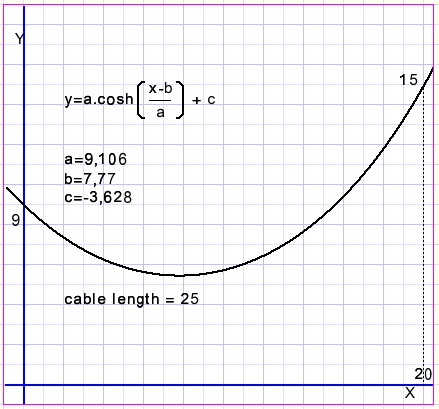Hanging cablesProblem

A non-elastic but flexible cable of a fixed length is attached at two points.
Which function describes the points on such an arc?

Given are the length L of the cable and the coordinates of the two ends: (x1,y1) and (x2,y2)

The basics

For a proof of the next functions please refer to Wikipedia, using "catenary" or "hanging cables" as keywords.
The general function describing the hanging cable arc is
y = a.cosh(x/a).....................where cosh(x) = (ex+e-x)/2
The length L1 of an arc from x=0 to x = x1 is
L1=a.sinh(x1/a).....................where sinh(x)=(ex-e-x)/2
The next pictures presents some arcs for different values of a: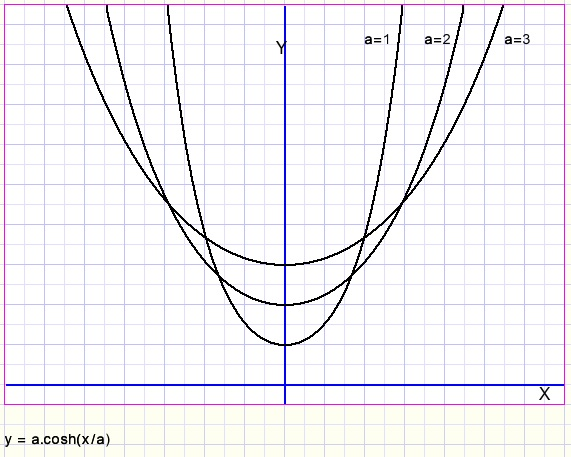We notice that the vertex is positioned on the Y-axis, which also is a line of symmetry.
A is the scaling factor, both horizontally and vertically.

For a start, our problem is this: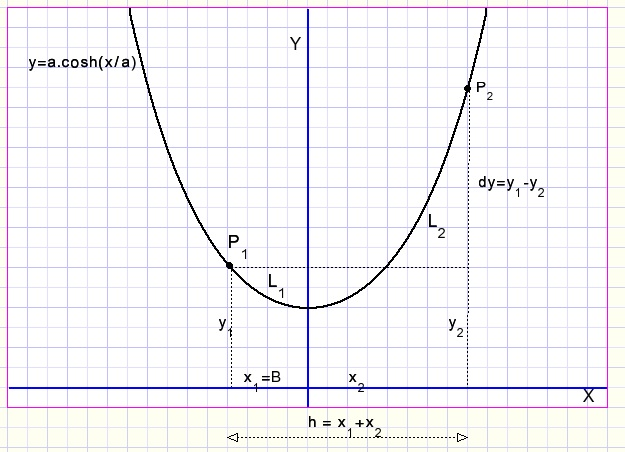Known values are the coordinates of points P1 and P2 and h = x1+x2
In this graph the minimum is y=a, but by adding a value C the function is shifted vertically without a change of L.

So what matters right now is the cable length L=L1+L2,
the difference dy = y2-y1 and the horizontal distance h=x1+x2.

This is what we know: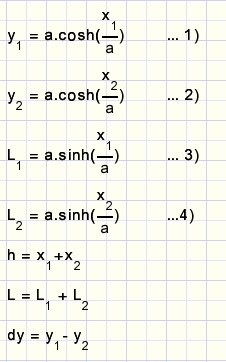First we want to know a.
We need to eliminate x1, x2, y1, y2 replacing them by the known values L, h and dy.

The calculation of a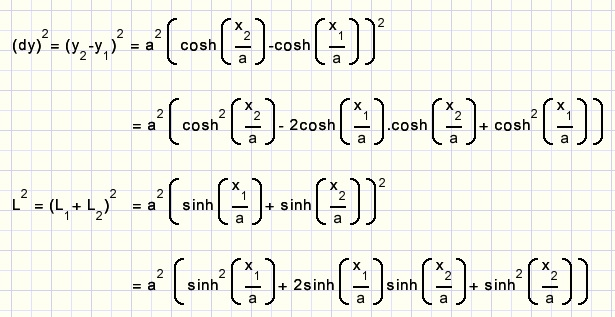Now we apply the rule: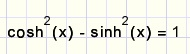and continue: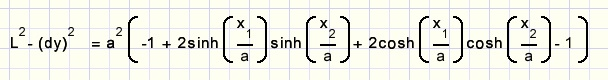Next we apply the rules: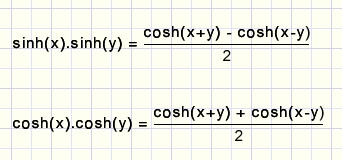and continue: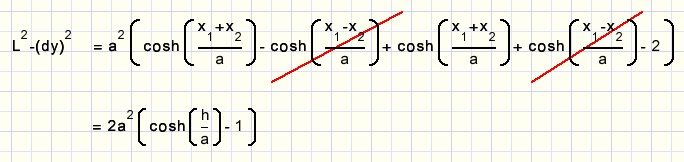One extra step by applying this rule: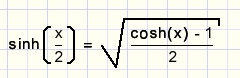and finally we arrive at: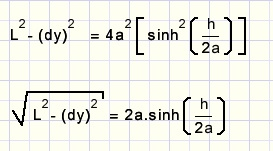In this equition a has to be solved numerically.

Calculation of b=x1

Knowing a, b=x1 may be calculated.

We apply the rule: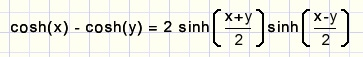and continue: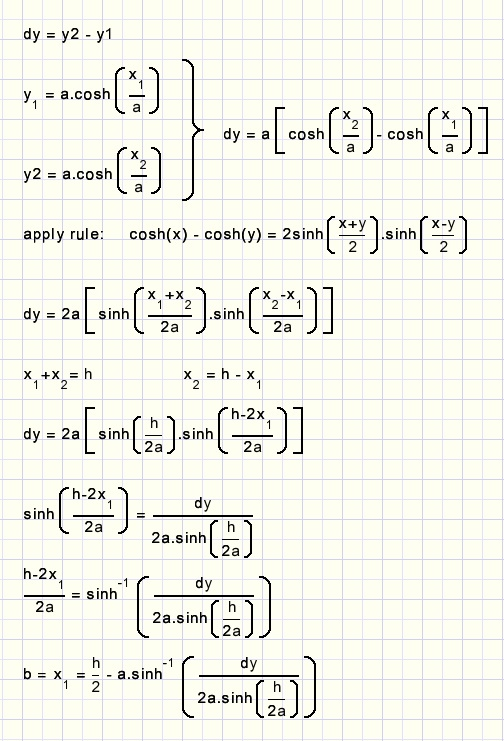In the final formula we want P1 on the Y-axis, so b = x1 is the horizontal shift required.
Note: this is done by replacing x by (x-b).
After this shift x1 = 0, x2 = h.
So far we have only used dy, not y1 and y2.
The minimum of the curve is a above the X-axis.

To make the curve hit points P1 and P2 a vertical shift is required.
Final formula:Calculation of c

Knowing a,b and y1 directly calculates c: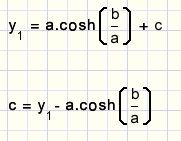Delphi program

In this program h (horizontal distance of points) = 20.
```procedure calculateArc(var A,B,C : double; y1,y2: double);
//given: linelength, (0,y1) , (20,y2)
//calculate formula y = A*cosh((x-B)/A) + C
var a1,a2,QLV,V,dy : double;
begin
a1 := 1;
a2 := 100;
dy := y1-y2;
QLV := 0.5*sqrt(sqr(linelength)-sqr(dy));
repeat
A := 0.5*(a1+a2);
V := A*sinh(10/A);
if V > QLV then a1 := A;
if V < QLV then a2 := A;
until abs(QLV - V) < 0.0001;
B := A*arcsinh(dy/(2*A*sinh(10/A))) + 10;
C := y1 - A*cosh(B/A);
end;
```
a is solved numerically by clamping between a1 and a2.
Note: the uses clause must include "math".